# 26th INTERNATIONAL MATHEMATICAL OLYMPIADFinland July 1–11 1985

## Introduction

38 countries participated, Luxembourg, Mexico and Venezuela were absent. China (2), Iceland (2) and Iran (1) appeared for the first time and India sent an observer. The other teams with less than the maximum 6 competitors were Italy (5), Kuwait (5), Spain (4) and Tunis (4).

Thus there were 209 competitors from 38 different countries. About a dozen were girls.

## The Choice of Problems

On June 29, two days before the teams arrived, the leaders met in Heinola as a Jury in order to decide the six problems of the competition. Several weeks before, each country had sent up to 5 problem-proposals to Finland. From these a selection of 6 first choices and further second choices had been made by the Finnish problem-selector for the Jury to consider.

In recent years some countries have felt that the range of mathematical knowledge required was too limited and the Finnish problem-selector had asked for a wider range of proposals. Three of the five problems submitted by G.B. involved knowledge of coordinate geometry applied to 1) plane similarity transformations, 2) the constant sum of focal distances in an ellipse and 3) two versions of a partitioning problem, one couched in the language of probability. None of these was selected for the consideration of the Jury. One ‘traditional’ G.B. problem was a second choice and the other was later produced when other geometry problems had been discarded, and finally selected as Question 1.

After the problem-papers had been decided, the problem-selector issued a pamphlet containing all proposals received with their solutions. It is evident from these proposals and discussion in the Jury that although some are in favour of reducing school geometry, particularly 3-D, and a few for introducing items in newer school syllabuses, most are content with the status quo.

Leaders come to the IMO having spent time (in some cases considerable time) training their teams and they are not going to vote for problems which seem to them outside the unwritten IMO syllabus. Should perhaps notice be given by host countries that proposals involving say complex numbers, coordinate geometry applied to curves and surfaces other than the circle and sphere, probability, etc. etc., will be accepted, or is this not worth while because such proposals will be voted down by the Jury?

The tradition is that the first and last of the 3 questions set on each of the two days should be ‘easy’ and ‘difficult’ respectively. Q3 proved to be the most difficult but it was fair in the sense that familiarity with binomial coefficients is to be expected and the difficulty lay in constructing a proof.

On the other hand Q6 involved the idea of nested intervals – two sequences converging to the same limit from above and below. It was a very nice question but comparatively easy for those whose approach to analysis starts after school with such ideas rather than as in G.B. with ‘engineering’ calculus in school. The U.S. leader had done no preparation in analysis of this sort in his 3-week training session and attributed the U.S. success (40/42) in this question to the fact that many U.S. universities hold week-end conferences for promising youngsters and a favourite lecture is on first year analysis, strange functions, etc.

Many of the leaders are university lecturers or professors in pure mathematics and much against ‘calculus’ being done in schools where the intricacies of limiting processes cannot be ‘properly’ explained. No doubt they expected that such ideas might be known or discovered by the abler pupils in their teams, as I did (cf. Area under a curve lying between the sum of upper and lower rectangular areas).

Of the two geometry questions Q1 and Q5, Q1 could be solved (inelegantly no doubt) by almost any sensible approach (e.g. using trigonometry). Q5 was chosen after an excellent 3-D polyhedron problem had been rejected and a substitute on vectors rejected as too easy. Eventually Q5 with its unnecessarily hard official solution was selected. (In fact both Q1 and Q5 were solved by G.B. competitors using no more than angle properties of quadrilateral and circle). From table 2, it can be seen that some leading countries find geometry difficult.

Q2 (combinatorics) and Q4 (prime factorisation and the pigeonhole principle) were both accepted without controversy.

## The Competition

On July 4 and 5 the competition (4½ hours each morning) took place in Joutsa where the deputies and teams had been housed since July 1. On July 5 the leaders left Heinola for Joutsa and were no longer held incommunicado from their teams. Deputies and leaders marked and submitted the scripts for each question to the Finnish co-ordinators for that question. The size of IMO is now such that each question has two pairs of co-ordinators and two days passed before all co-ordination was ended. Table 1 shows the British competitors, their marks and prizes and Table 2 shows the marks per question of those 14 countries whose total marks exceeded 100.

The remaining countries are those named in paragraph 1 and the following: Brazil, Israel, Austria, Cuba, Netherlands, Greece, Yugoslavia, Sweden, Mongolia, Belgium, Morocco, Colombia, Turkey, Algeria, Norway, Cyprus and Finland.

## Table 1

Question123456TotalPrize
C. KilgourKing’s School, Gloucester70022112
J. LongleyPortsmouth Grammar School77020016III
M. MooreManchester Grammar School77300017III
M. RichardsMillfield School77177332II
A. SelbyCity of London School77020117III
I. StarkWinchester College77247027II
4235617165121
Average mark for a team of six2522 515111290

George Megyesi of Atlantic College, Glamorgan was chosen for the Hungarian team. He shared first prize in the British Mathematical Olympiad with Matthew Richards and got one of the two perfect scores (Ian Stark got the other) in our multiple-choice National Mathematics Competition. His mark in IMO was 38. Only 2 competitors (another Hungarian and a Romanian) did better, each getting the maximum 42.

## Table 2

Prizes
Question123456TotalIIIIII
Romania42421635323420133
United States28383430104018024
Hungary423117302325168222
Bulgaria42351022332316523
Vietnam4235225281214414
Soviet Union284221141520140122
West Germany25411626823139114
East Germany36351119102513633
France1542124133012523
10 Great Britain423561716512123
11 Australia34337191014117112
12=Czechoslovakia2231115142210531
14 Poland333514111710114

The remaining 24 countries scored less than 100 marks,
Belgium (total score 60) had a first prize winner.
Soviet Union’s first prize winner was a girl.

## The British Team

Their overall performance was disappointing. Matthew Richards was unlucky not to get a first prize. First, second, third and no prizes have commonly been given in the ratio 1:2:3:6. This year only 14 first prizes were given and Richards had the highest mark not to get a first prize.

There were several ingenious attempts and David Monk spent some time trying to ‘rescue’ solutions which proved to be too unsound for the co-ordinators to allow few if any marks.

We noticed rather more obscurity in presentation than in previous years. Some of the team obviously had an off-day and did not do themselves justice. Two British solutions were put up for special prizes but were not considered ‘special’ or striking enough. No special prizes were given this year.

The six British competitors were a very pleasant lot. They got on well with each other and with their English-speaking rivals. They enjoyed themselves and did not let their disappointments get them down.

## Non-Mathematical Matters

We are very grateful to our Finnish hosts, not only for the excellent organisation of the Competition, but for the programme of sightseeing, informal games, football, tennis, boating, swimming, saunas, picnics and concerts, all contributing opportunities for ‘friendship between the young of 38 nations’. Teams had young Finnish students as attachés and our attachée was very helpful indeed looking after our general interests, keeping us up to the mark and overcoming language difficulties.

On the last evening various countries contributed to an informal variety show. Our team sang two Swan-songs – ‘The English are Best’ and ‘Mud, mud, Glorious Mud.’

## The Future

At the last meeting of the Jury, John Hersee, Secretary of the IMO Site Committee, told us that future host countries were – 1986, Poland: 1987, Cuba: 1988, Australia. Sweden and West Germany were among those to follow. The difficulties in wide mathematical disparity and in the organising of ever-increasing numbers were discussed. Surprisingly, the increasing cost to future host countries was not thought too heavy by most of the five leaders concerned above. They seemed confident that governmental, industrial and academic support will be readily forthcoming.

Robert Lyness.

## FIRST DAYJoutsa    July 4, 1985

The name of the country proposing the question has been added at the end of each question. On the papers given to competitors these names were not given.

1. A circle has centre on the side AB of the cyclic quadrilateral ABCD. The other three sides are tangent to the circle. Prove that AD + BC = AB.

Great Britain.

2. Let n and k be given relatively prime natural numbers, 0 < k < n. Each number in the set M = { 1, 2, ..., n-1 } is coloured either blue or white. It is given that

1. for each, both i and n-i have the same colour, and
2. for each,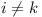, both i and |i - k| have the same colour.

Prove that all numbers in M must have the same colour.

Australia.

3. For any polynomial P(x) = a0 + a1x + ... + akxk with integer coefficients, the number of coefficients which are odd is denoted by w(P). For i = 0, 1, 2, ... let Qi(x) = (1 + x)i. Prove that if i1, i2, ..., in are integers such that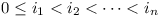, then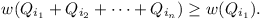Netherlands.

Time allowed: 4½ hours
Each problem is worth 7 points.

## SECOND DAYJoutsa    July 5, 1985

1. Given a set M of 1985 distinct positive integers, none of which has a prime divisor greater than 26. Prove that M contains at least one subset of four distinct elements whose product is the fourth power of an integer.

Mongolia.

2. A circle with centre O passes through the vertices A and C of triangle ABC, and intersects the segments AB and BC again at distinct points K and N, respectively. The circumscribed circles of the triangles ABC and KBN intersect at exactly two distinct points B and M. Prove that angle OMB is a right angle.

Soviet Union.

3. For every real number x1, construct the sequence x1, x2, ... by setting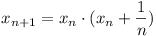for each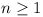. Prove that there exists exactly one value of x1 for which 0 < xn < xn+1 < 1 for every n.

Sweden.

Time allowed: 4½ hours
Each problem is worth 7 points.

## SOLUTIONS

These solutions are based on official solutions and will not be issued as they stand to schools who apply for IMO 1985 papers to the Mathematical Association.

1. Let the points of tangency be E, F and G as shown in the figure. By elementary trigonometry, we obtain

 AD + BC = r(cot y − cot 2x) + r(cot x − cot 2y) = r(cot y − cot 2y) + r(cot x − cot 2x) = r csc 2y + r csc 2x = AO + OB = AB.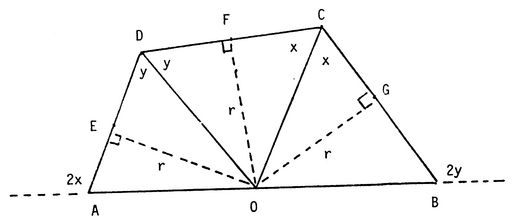2. The result is trivially true for n = 2. Suppose that the result is false and consider the smallest n ≥ 3 for which there exists a non-constant two-colouring of M = { 1, 2, …, n−1 } satisfying (i) and (ii) for some O < k < n. In this example, let j be the least element of M which is coloured differently from element 1. There are three cases to consider.

1. j > k. This is impossible since j and jk are required to have the same colour.

2. j = k. The elements nk+1, …, n−1 must have the same colour as element 1 but k, 2k, … must have the opposite colour. This implies that nk is a multiple of k. But this is impossible since k = j > 1 and (nk) = 1.

3. j < k. Write n = qk + r, 0 < r < k, and note that (kr) = 1. Consider the induced colouring of M' = { 1, 2, …, k−1 } and note that it has the following properties:

1. For each i ∈ M', both i and ki have the same colour.
2. For each i ∈ M', i ≠ r, both i and |i − r| have the same colour.

To see (ii), note that if r > i then i, ni, nki, …, ri is a monochromatic sequence, and if r < i then ir, k+ri, …, ni, i is a monochromatic sequence. Since the two-colouring of M' satisfies (i) and (ii) and is non-constant (i and j have opposite colours), we have reached a contradiction to our choice of n as providing a minimal counterexample.

3. The proof is by induction on in. For in = 0 or 1 the result is trivial. Let R and S denote polynomials with integer coefficients. The following facts will be useful.

1. If k = 2m then (1 + x)k ≡ 1 + xk (mod 2),
2. If deg R < k then w(R + xk S) = w(R) + w(S),
3. |w(R) - w(S)| ≤ (R + S) ≤ w(R) + w(S) (triangle inequality).

Let in > 1 be given and write k = 2min < 2m+1. There are two cases to consider.

1. i1 < k. Write Qi1 + … + Qin as R + (1 + x)k S where R = Qi1 + … + Qir and deg R, deg S < k. Then

 w(Qi1 + … + Qin) = w(R + S + xk S) (a) = w (R + S) + w(S) (b) ≥ w(R) (c) ≥ w(Qi1) (induction hypothesis).
2. i1k. Write Qi1 = (1 + x)k R and Qi1 + … + Qin = (1 + x)k S. Then deg R, deg S < k and we have

 w(Qi1 + … + Qin) = w(S + xk S) (a) = 2 w(S) (b) ≥ 2 w(R) (induction hypothesis) = w(R + xkR) (b) = w(Qi1) (a).
4. Let p1 = 2, p2 = 3, …, p9 = 23. For each element of M there is a corresponding vector (x1x2, …, x9) where xi = 0 if, in the prime factorization of the element, pi has an even exponent and 1 if the exponent is odd. By the pigeonhole principle, any subset of 29 + 1 = 513 elements of M contains two distinct elements with the same exponent vector, i.e. two distinct elements whose product is a perfect square. It follows that M contains at least 737 pairs of distinct elements where the product of each pair is a perfect square.* Consider the square roots of these products. Again the prime factors are limited to p1, …, p9 and all we need are 513 to be sure that two have a product which is a perfect square. We thus find aibi = ci2 and ajbj = cj2 where ai, aj, bi, bj are elements of M and cicj = d2, i.e. aibiajbj = d4.

* To see this: Split the 1985 given integers arbitrarily into sets A, B, C containing initially 1472, 513 and 0 integers respectively. “Take a pair of integers with the same vector from B and place the pair in C. Transfer any 2 integers from A to B. Then B has 513 integers again and must contain 2 integers with the same vector.”

Repeat the instructions in the sentences in quotation marks until A is empty. There will be then 737 pairs in C.

5. The common chords of the three pairs of circles are concurrent at their radical centre. Calling P the radical centre, quadrilateral PMNC is cyclic (because ∠PMN = ∠BKN = ∠NCA). Thus

BM.BP = BN.BC = BO2r2
PM.PB = PN.PK = PO2r2,

where r is the circumradius of triangle AKC. Hence

 PO2 − BO2 = BP (PM - BM) = (PM + BM)(PM − BM) = PM2 − BM2.

This proves that 0M is perpendicular to BM.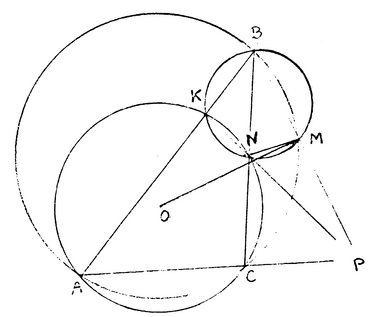6. Let x1 = x. Then x = Pn(x) where Pn is a polynomial of degree 2n−1 with positive coefficients. Note that Pn is increasing and convex for x ≥ 0. Observing that the condition xn+1 > xn is equivalent to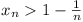, the problem can be reformulated as follows: there is a unique positive real number t such that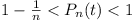for all n ≥ 1. Since Pn is increasing for x ≥ 0 and Pn(0) = 0, it follows that for each n there are unique values for an and bn such that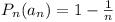and Pn(bn) = 1 respectively. Note that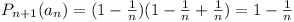and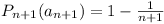. Since Pn+1 is increasing, it follows that an < an+1. Similarly,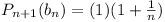and Pn+1(bn+1) = 1 so bn+1 < bn. It follows that [anbn] is a nested sequence of closed intervals and its intersection is non-empty. Since Pn is convex for x ≥ 0 and Pn(0) = 0, we find that Pn(x) ≤ x/bn, 0 ≤ x ≤ 1, in particular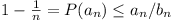. Together with the fact that 1 = b1 > b2 > b3 > … this means that bn − an ≤ 1/n for all n. Consequently, there is only one point t which belongs to all the intervals. This point satisfiesfor all n and it does so uniquely. For any point x ≠ t, either x < an or bn < x for sufficiently large n meaning that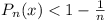or else Pn(x) > 1.